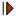# Eight Point Circle Theorem: Quadrilateral. Interactive Geometry Software.

Hint to interact with the figure below: Click the red button () on the figure to start the animation. Move the points A, C, and D to explore the figure. Press P and click the left mouse button to start the step by step construction, help.

Proposition
Given a quadrilateral ABCD with perpendicular diagonals AC and BD. The four midpoints E,F,G, H of each sides, and the four feet E',F',G',H' of perpendiculars from the midpoints to the opposite sides all lie on a circle called the eight-point circle.

The center O of the eight-point circle is the centroid or gravity center of quadrilateral ABCD.

EFGH is a rectangle. See Varignon parallelogram.

The eight point circle was first discovered by Louis Brand of Cincinnati in 1944. This theorem can be useful in proving the far more famous nine point circle theorem. Reference: The American Mathematical Monthly, Vol. 51, No. 2 (Feb., 1944), pp. 84-85

Dynamic Geometry: You can alter the figure dynamically in order to test and prove (or disproved) conjectures and gain mathematical insight that is less readily available with static drawings by hand.

This page uses the TracenPoche dynamic geometry software and requires Adobe Flash player 7 or higher. TracenPoche is a project of Sesamath, an association of French teachers of mathematics.

Instruction to explore the dynamic figure:

• Animation. Click the red buttonto start/stop animation

• Manipulate. Drag points A , C, and D to change the figure.

• Step-by-Step construction. Press P and click the left mouse button on any free area of the figure above to show the step-by-step bar and click 'Next Step' button () to start the construction step-by-step:Hide the step-by-step bar by using again the combination P + click left mouse.

 Home | Geometry | Dynamic Geometry | TracenPoche Index | Email | By Antonio Gutierrez Last updated: August 1, 2009